Latest Banking jobs   »

# Reasoning Quiz For RBI Grade B Phase 1 2023-17th May

Directions (1-4): Study the following information to answer the given questions.
A person starts moving from Point A towards east direction to point B. After walking 5km to reach point B he turns to the left and walks 5km to reach point C. From there he turns to his left and walks 8 km and reached point D. After reaching to point D he turns to the south direction and walks 10km and reached to point E. Then he again turns to his left and walks 6km to reach point F. Finally, he turns to left and walks 3km and reach to point G.

Q1. What is the shortest distance between point A and point G?
(a) 3 km
(b) 8 km
(c) 10 km
(d) 5 km
(e) None of these

Q2. Point C is in which direction with respect to point E?
(a) North-East
(b) South
(c) East
(d) South-West
(e) None of these

Q3. If the person further travels 5km from point G to reach point H then Point B is in which direction with respect to point H?
(a) North-East
(b) South
(c) East
(d) South-West
(e) Can’t be determined

Q4. How far is point C with respect to point A?
(a) 3√2 km
(b) 7√5 km
(c) 11√3 km
(d) 5√2 km
(e) None of these

Directions (5-7): Study the following information and answer the given questions
Point Y is 12m to the North of Point X. Point Z is 8m to the East of Point W. Point M is 3m to the South of Point W. Point M is 4m to the West of Point N. Point Z lies exactly between Point X and Point Y.

Q5. In which direction is Point X with respect to Point N?
(a) North
(b) Northeast
(c) South
(d) Southwest
(e) None of these

Q6. If Point V lies 6m to the North of Point W then how far is Point V from Point Y?
(a) 6m
(b) 8m
(c) 10m
(d) 12m
(e) Cannot be determined

Q7. What is the shortest distance between Point Z and Point N?
(a) 3m
(b) 4m
(c) 5m
(d) 6m
(e) None of these

Directions (8-9): Study the following information carefully to answer the given questions below.
Point L is 3m to the west of point K. Point M is 2m north of point K. Point N is 4m east of point M. Point O is 2m south of point L. Point J is 3m east of point O.

Q8. Which among the following points are in a straight line?
(a) O, J, K
(b) M, N, L
(c) L, K, J
(d) M, K, J
(e) L, K, M, N

Q9. In which direction is point N with respect to point O?
(a) North-east
(b) South-west
(c) North-west
(d) South
(e) None of these

Directions (10): Study the following information carefully and answer the given questions.
Shyam starts travelling in north direction from point A. After travelling 3km he reached point B. From there he takes a right turn and travel 6km. Then he reached point C and from there he turned towards left and walk 2km and reached point D. From there he turned towards left and after walking 3km, he finally stops at point E.

Q10. What is the shortest distance between point E and point A?
(a) √32
(b) √34
(c) 3√2
(d) 2√3
(e) None of these

Solutions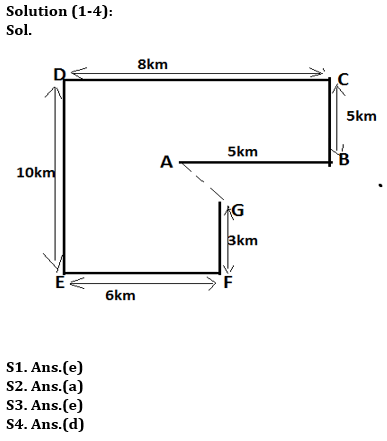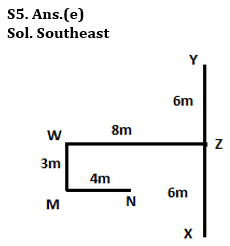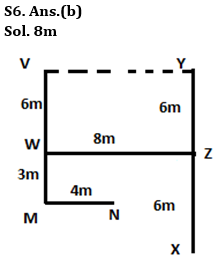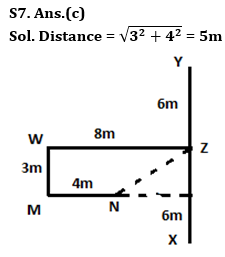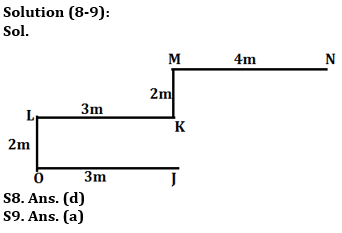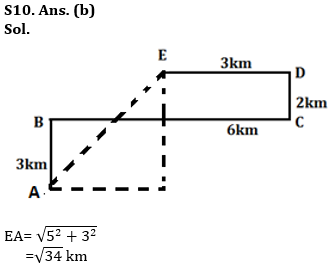## FAQs

### How many sections are there in the RBI Grade B Phase 1 Exam?

There are 4 sections in the RBI Grade B Phase 1 Exam i.e. English Language, General Awareness, Quantitative Aptitude & Reasoning.

#### Congratulations!Union Budget 2023-24: Free PDF